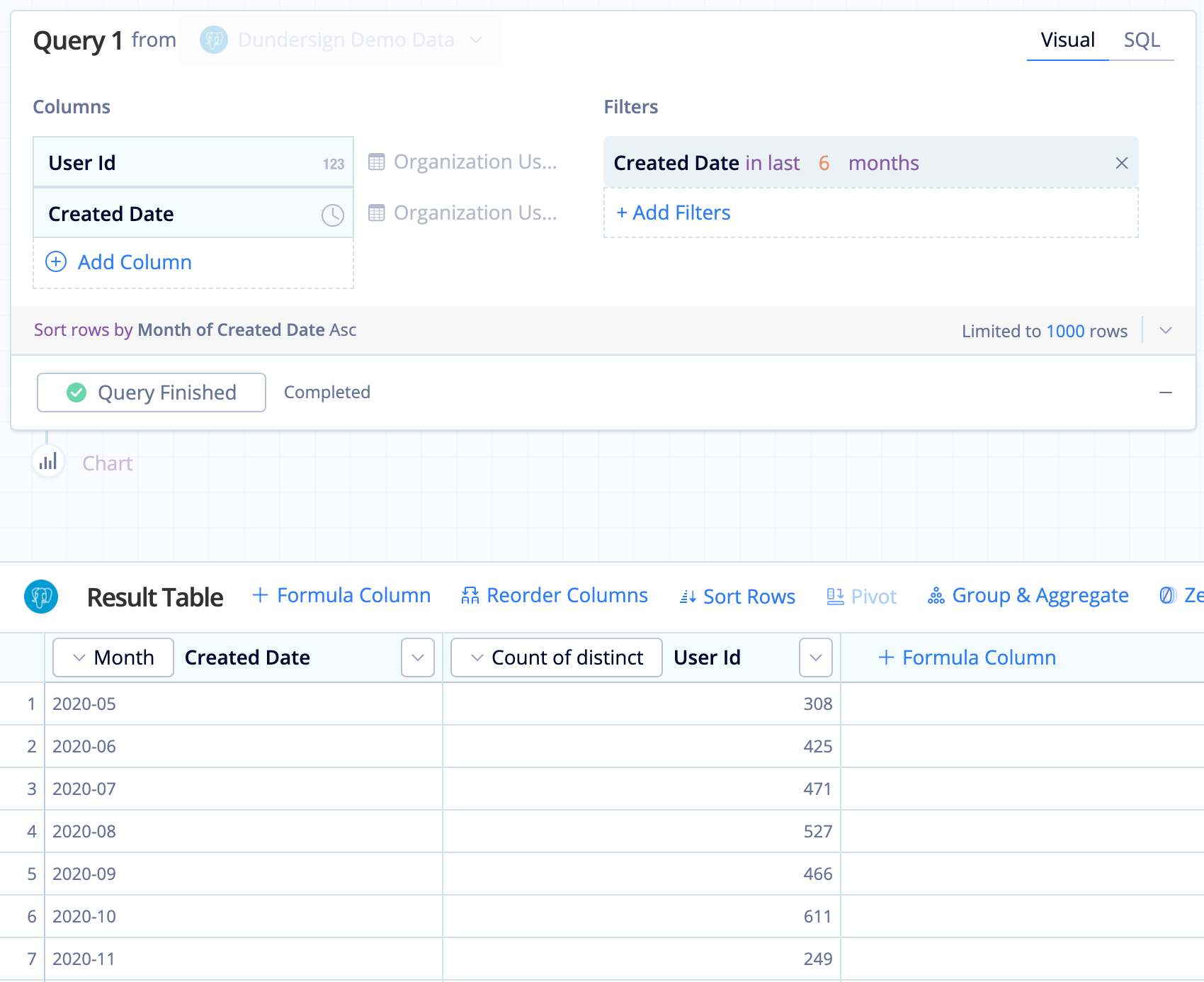# Count distinct totals and percentages in Visual SQL

In this example, we create a Table chart using our Dundersign Demo Data data source to compare the number of distinct users per month over the last six months and include the percentage each month’s count contributes to the six-month total.

1. Create the base query:
1. Add the User Id and Created Date columns from the Users table to the Columns section.
2. Also add the Created Date column to the Filters section, filtering for the data from the last six months.

We chose the last N months filter operator with a value of 6 months.

3. Change the time bucket of Created Date to Month.
4. Click Run Query.2. Next, add a Formula Column, use the Ratio of Total Guided Formula, and select the User Id column. Each row in this new column will show the percentage that month’s user count contributes to the total six-month user count.

3. Rename the “Ratio of Total User Id” column to % of Total for a bit more clarity.

4. To add the finishing touches to the Table chart, open the chart settings by clicking the Gear.
1. If you want to see the total six-month user count in your Table chart, select Show row aggregation for numeric columns from the General settings tab. By default, the row aggregation is shown as the bottommost row of the table, but you can opt to make it the topmost row by selecting Show aggregation row at the top.
2. Lastly, you need to make the % of Total actually show percentages! Go to the Columns settings tab and click + Add New Formatting Rule. Select % of Total for the Format Column and Percentage for the Data Format. You can change the precision with the Number Formatting field; by default, the numbers are rounded to the nearest integer.

Note how the total is counted for both User Id column and the % of Total column. If you only need the total count of a single column, you can use the Aggregation Guided Formula with the SUM aggregation function.